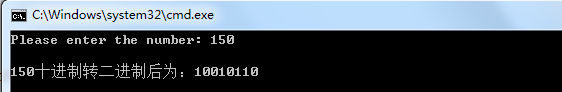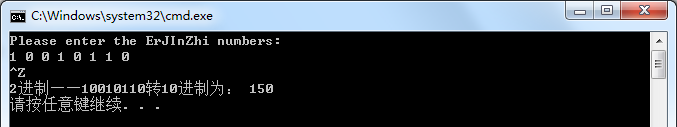• 主要介绍了python十进制二进制转换方法(含浮点数)，小编觉得挺不错的，现在分享给大家，也给大家做个参考。一起跟随小编过来看看吧
• 适用于将二进制转换十进制，A为十进制，B为二进制。{A,B}每次左移一位，判断A的每四位是否＞4，若大于四则+3，否则保持不变；B为多少位二进制数则左移多少次。...代码为32位二进制转换十进制数。
• 主要介绍了C语言十进制二进制代码实例,并且转换后会统计二进制1的个数,实例简单明了,需要的朋友可以参考下
• 二进制十进制，首先讲一下“权重”的概念 数字中某位的权重：2的（该位所在的位数（从右至左）-1）次方 比如：10 0的权重为：2^(1-1)=1 1的权重为：2^(2-1)=2 二进制十进制：数字中所有位*本位的权重...
• 主要介绍了Python实现的十进制小数与二进制小数相互转换功能,结合具体实例形式详细分析了二进制十进制相互转换的原理及Python相关实现技巧,需要的朋友可以参考下
• 下面小编就为大家带来一篇浅谈十进制小数和二进制小数之间的转换。小编觉得挺不错的现在就分享给大家，也给大家做个参考。一起跟随小编过来看看吧
• 主要介绍了C语言用栈实现十进制转换二进制的方法,结合实例形式分析了C语言栈的定义及进制转换使用技巧,需要的朋友可以参考下
• 二进制转换十进制 C++实现二进制转换十进制 十进制与二进制之间的转换 十进制转换二进制 十进制对2整除，得到的余数的倒序即为转换而成的二进制 十进制转换二进制 C++实现十进制转换二进制 主函数main.cpp为...

欢迎关注原创公众号 【计算机视觉联盟】，回复 【西瓜书手推笔记】 可获取我的机器学习纯手推笔记！
直达笔记地址：机器学习手推笔记（GitHub地址）

目录
十进制与二进制之间的转换
十进制转换二进制
C++实现十进制转换二进制
二进制转换十进制
C++实现二进制转换十进制

十进制与二进制之间的转换
十进制转换二进制
十进制对2整除，得到的余数的倒序即为转换而成的二进制

十进制转换二进制

C++实现十进制转换二进制
主函数main.cpp为：
#include<iostream>
#include<fstream>
#include<string>
#include<vector>
#include<cmath>
using namespace std;
int main()
{

int N;
cout << "Please enter the number: ";
cin >> N;
cout << endl;

//十进制转二进制的声明和调用
void  convert_10_to_2(int num);
convert_10_to_2(N);
}
十进制转换为二进制函数为：
#include<iostream>
#include<fstream>
#include<string>
#include<vector>
#include<cmath>
using namespace std;
void convert_10_to_2(int num)
{
vector<int> ivec;
int Num = num;  //存储原数据
//对原数据循环求除以2的余数,并存储在vector对象中
while (num != 0)
{
ivec.push_back(num % 2);
num = num / 2;
}

//对求得的余数倒序排序并存储在vector中，求得二进制
vector<int>::size_type sz = ivec.size();
vector<int> ivec2;
for (vector<int>::size_type index = 0; index != sz; ++index)
ivec2.push_back(ivec[sz - 1 - index]);

//输出十进制转换为二进制的数
cout <<Num  << "十进制转二进制后为：";
for (auto item : ivec2)
cout << item;
cout << endl<<endl;
}
运行结果为：二进制转换十进制
将二进制从右往左从零次幂开始乘以二进制数并求和

二进制转换为十进制

C++实现二进制转换十进制
C++主函数为：
#include<iostream>
#include<fstream>
#include<string>
#include<vector>
#include<cmath>
using namespace std;
int main()
{
double convert_2_to_10();
convert_2_to_10();
return 0;
}
二进制转十进制函数为：
#include<iostream>
#include<fstream>
#include<string>
#include<vector>
using namespace std;
double convert_2_to_10()
{
//输入二进制数并存储在vector变量ivec2中
cout << "Please enter the ErJInZhi numbers:  " << endl;
int n;
vector<int> ivec2;
while (cin >> n)
{
ivec2.push_back(n);
}

//二进制转十进制计算
double sum = 0, times;
vector<int>::size_type sz = ivec2.size();
for (vector<int>::size_type index = 0; index != sz; ++index)
{
times = pow(2, sz - 1 - index);
sum += ivec2[index] * times;
}

//输出转化后的结果
cout << "2进制——";
for (vector<int>::size_type index = 0; index != sz; ++index)
cout << ivec2[index];
cout << "转10进制为： ";
cout << sum << endl;
return 0;
}
运行结果：（输入二进制时要以空格区分）展开全文C++
• 一，十进制（decimal system）转换函数说明 1，十进制二进制 decbin() 函数，如下实例 echo decbin(12); //输出 1100 echo decbin(26); //输出 11010 decbin (PHP 3, PHP 4, PHP 5) decbin — 十进制转换二进制 ...
• 本程序用C语言实现了二进制十进制的相互转换，适于C语言初学者作参考
• 题目内容：将十进制整数转换二进制数。 输入描述：输入数据中含有不多于50个的整数n(-231”，再然后输出二进制数。每个整数n的输出，独立占一行。 题目分析：将某个数从十进制转为二进制的具体方法是，该数对2取余...
• 本文主要讲了单片机二进制十进制程序代码，希望对你的学习有所帮助。
• 本文给大家介绍的是一则使用C++实现读入二进制数并转换十进制输出的代码，实现起来其实非常简单，C++本身就提供了二进制类库的，大家看代码吧，简单又实用。
• 该项目（双转换）为IEEE double提供了二进制十进制和十进制二进制例程。 该库包含从V8 JavaScript引擎中提取的高效转换例程。 该代码已经过重构和改进，因此可以在其他项目中更轻松地使用。 在double-conversion/...C++
• 主要介绍了Python中不同进制互相转换,本文讲解了二进制、八进制、十进制和十六进制的相与转换实现代码,需要的朋友可以参考下
•matlab
• 二进制转换十进制 1. 将十进制转换为二进制： 思路：对十进制的数进行除2取余法： /** * 讲10 进制转化为二进制 * @param de ：待转换的十进制 * @return ：转换后的二进制（string） */ public static ...


将十进制转换为二进制将二进制转换为十进制

1. 将十进制转换为二进制：

思路：对十进制的数进行除2取余法：

    /**
* 讲10 进制转化为二进制
* @param de ：待转换的十进制
* @return   ：转换后的二进制（string）
*/
public static String Decimal2Binary(int de){
String numstr = "";
while (de>0){
int res = de%2; //除2 取余数作为二进制数
numstr = res + numstr;
de = de/2;
}
return  numstr;
}

2. 将二进制转换为十进制

思路：对二进制从后往前数第i位上的数进行乘以2的i-1 次方；

    /**
* 将二进制转换为10进制
* @param bi ：待转换的二进制
* @return
*/
public  static  Integer Biannary2Decimal(int bi){
String binStr = bi+"";
Integer sum = 0;
int len = binStr.length();
for (int i=1;i<=len;i++){
//第i位 的数字为：
int dt = Integer.parseInt(binStr.substring(i-1,i));
sum+=(int)Math.pow(2,len-i)*dt;
}
return  sum;
}

完整代码：


import java.awt.*;
import java.util.Scanner;

/**
* Created by chen on 2020/7/12.
*/
public class Test {
public static void main(String args[]) {

//testD2B();
//testB2D();
}
/**
* 讲10 进制转化为二进制
* @param de
* @return
*/
public static String Decimal2Binary(int de){
String numstr = "";
while (de>0){
int res = de%2; //除2 取余数作为二进制数
numstr = res + numstr;
de = de/2;
}
return  numstr;
}

/**
* 将二进制转换为10进制
* @param bi
* @return
*/
public  static  Integer Biannary2Decimal(int bi){

String binStr = bi+"";

Integer sum = 0;
int len = binStr.length();

for (int i=1;i<=len;i++){
//第i位 的数字为：
int dt = Integer.parseInt(binStr.substring(i-1,i));
sum+=(int)Math.pow(2,len-i)*dt;
}
return  sum;
}

public static void testB2D(){
while (true){

System.out.println("Pleace input a Binary num:");
Scanner sc = new Scanner(System.in);
int binary = sc.nextInt();

int out = Biannary2Decimal(binary);

System.out.println("The Decimal num is :" + out);

System.out.println("输入0 结束，输入1 继续");
sc = new Scanner(System.in);

if (sc.nextInt()==0){
break;
}
}
}

public static void testD2B(){
while (true) {

System.out.println("Pleace input a int Decimal num:");
Scanner sc = new Scanner(System.in);
int num = sc.nextInt();
String numofBinary = Decimal2Binary(num);

System.out.println("The Binary num is :" + numofBinary);

System.out.println("输入0 结束，输入1继续");
sc = new Scanner(System.in);

if (sc.nextInt() == 0) {
break;
}
}
}

}


展开全文java
• 十进制转换二进制数以及十六进数，代码完整可运行，对于初学者有用。
• C语言-顺序栈实现十进制转换二进制-八进制-十六进制
• 在Matlab中十进制转化为二进制矩阵，可以生成波形，比使用Matlab中自带的函数生成的char型结构更合理
• 此文档为《十进制小数和二进制小数相互转换》，让C++初学者更好的理解进制转换【独家出版，未经允许，禁止侵权】编程
• 主要实现二进制和十进制的数字转换的源代码，void CharToHex(char * dest, char * buffer , int len)中实现了字母间的二进制转换
• 将任意进制字符串转换十进制，如二进制，八进制，十六进制, 第二数数不写即为最常用的转换为整型十进制代码如下: parseInt(“11”, 2); // 3 2进制转10进制 parseInt(“77”, 8); // 63 8进制转10进制 ...
• 主要介绍了使用Python内置函数：bin()、oct()、int()、hex()可实现进制转换的一些用法,需要的朋友可以参考下...